StatLect

# Systems of linear equations and matrices

In this lecture we show how matrices and vectors can be used to represent and analyze systems of linear equations.## Systems of linear equations

A system oflinear equations inunknowns is a set of equations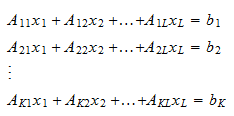whereare theunknowns, and(forand) and(for) are known constants.

## Solutions

The unknowns are the values that we would like to find. Solving a system of linear equations means finding a set of values forsuch that all the equations are satisfied. Such a set is called a solution of the system.

Example Define the systemIt is a system of 2 equations in 2 unknowns. A solution of the system iswhich can be verified by substituting these two values into the system: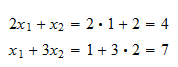In general, a solution is not guaranteed to exist. If it exists, it is not guaranteed to be unique. Therefore, the theory of linear equations is concerned with three main aspects:

• deriving conditions for the existence of solutions of a linear system;

• understanding whether a solution is unique, and how multiple solutions are related to each other;

• finding techniques that allow to find solutions of a linear system.

## Matrix representation of a system

The above system oflinear equations inunknowns can be represented compactly by using matrices as follows:where:

•is thevector of unknowns;

•is thematrix of coefficients, whose-th elementis the constant that multipliesin the-th equation of the system;

•is thevector of constants.

To understand how the representation works, notice thatis avector whose-th element is equal to the inner product of the-th row ofand, that is,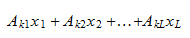Therefore,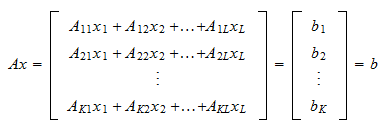Example The systemcan be represented aswhere thematrix of coefficients is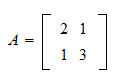thevector of unknowns isand thevector of constant terms is## Existence of solutions

By writing a system of linear equations in matrix form, we can easily provide general conditions for the existence of a solution.

Proposition The linear systemhas a solution if and only ifbelongs to the span of the columns of.

Proof

The productcan be interpreted as a linear combination of the columns of, with coefficients taken from. Therefore, the problem of solving the system is tantamount to finding a vector of coefficientsthat allows us to writeas a linear combination of the columns of. Butcan be written as a linear combination of the columns ofif and only if it belongs to their span.

## Uniqueness of the solution

We now give a general condition for the uniqueness of the solution.

Proposition If the linear systemhas a solution, then the solution is unique if and only if the columns ofare linearly independentProof

Let's first prove the if part. We have proved above that there is a solution if and only ifbelongs to the span of the columns of. If the columns ofare linearly independent, then they form a basis for their span. Furthermore, the representation of any vector of the span as a linear combination of the basis is unique. Therefore, if the columns ofare linearly independent, there is only one linear combination of them that givesas a result, that is, the solution of the system is unique. Let's now prove the only if part. We are going to prove that if the columns are not independent, then there is more than one solution. Letbe a solution, that isWhen the columns ofare linearly dependent, there exist a non-zero vectorthat satisfiesAs a consequence, there are infinite solutions becauseis a solution of the system for any scalar:## Multiple solutions

The following proposition about multiple solution holds.

Proposition If the linear systemhas a solution and the columns ofare not linearly independent, then there are infinite solutions.

Proof

See the previous proof.

## Solved exercises

Below you can find some exercises with explained solutions.

### Exercise 1

Find the matrix representation of the systemSolution

The system can be represented aswhere thematrix of coefficients is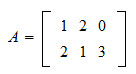thevector of unknowns isand thevector of constant terms is### Exercise 2

DefineWrite down the equations of the systemSolution

The two equations of the systems areThe book

Most of the learning materials found on this website are now available in a traditional textbook format.

Glossary entries
Share